# EU Long-Term Interest Rates, August 2019

Oct 3, 2019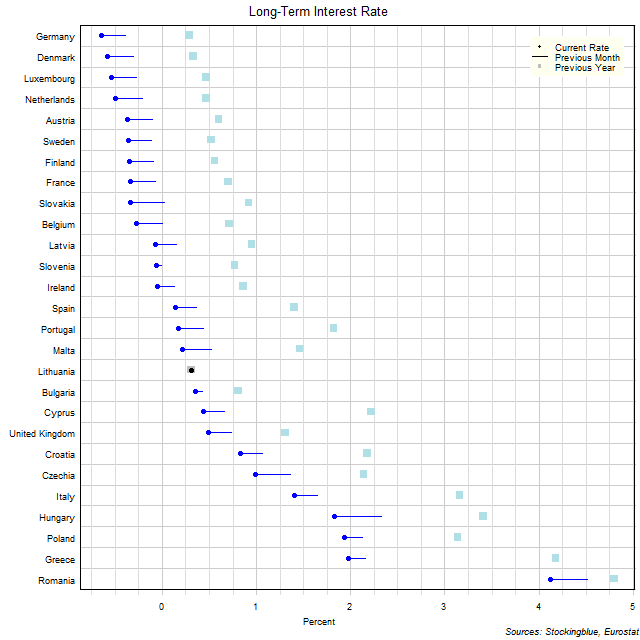The chart above shows the ten-year interest rate in each EU state as of August 2019, the change from the previous month, and the rate one year prior.  Thirteen states have a negative interest rate (up from nine last month and zero last year).

# EU Long-Term Interest Rates, July 2019

Sep 5, 2019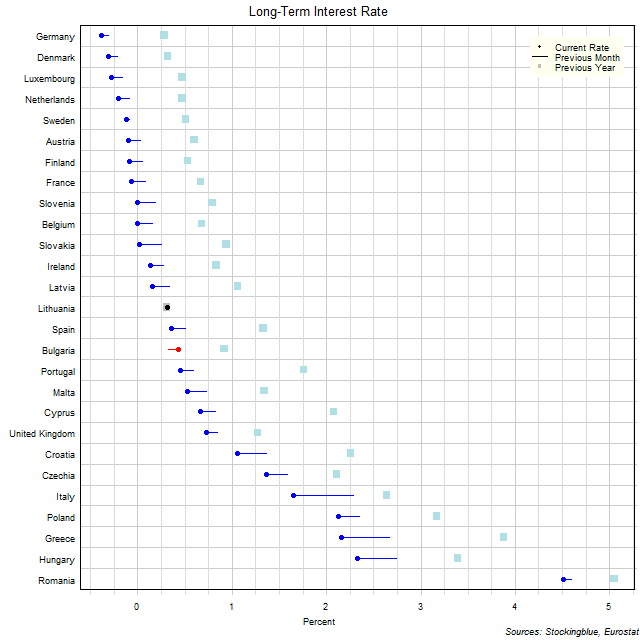The chart above shows the ten-year interest rate in each EU state as of July 2019, the change from the previous month, and the rate one year prior.  Nine states have a negative interest rate (up from five last month and zero last year).

# EU Long-Term Interest Rates, June 2019

Aug 8, 2019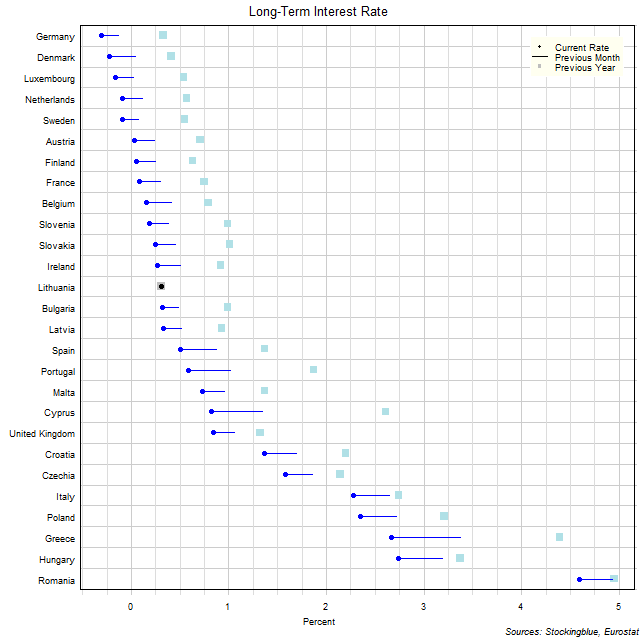The chart above shows the ten-year interest rate in each EU state as of June 2019, the change from the previous month, and the rate one year prior.  Five states have a negative interest rate.

# EU Long-Term Interest Rates, May 2019

Jul 4, 2019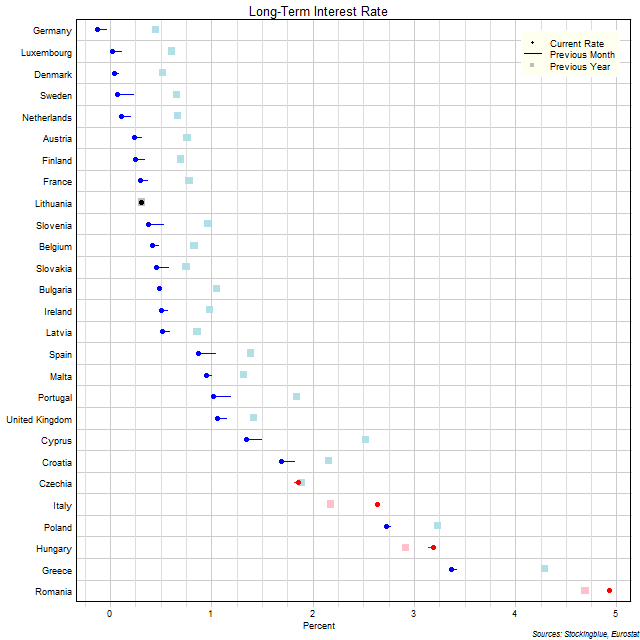The chart above shows the ten-year interest rate in each EU state as of May 2019, the change from the previous month, and the rate one year prior.  Germany continues to be the only state with a negative interest rate.

# EU Long-Term Interest Rates, April 2019

Jun 6, 2019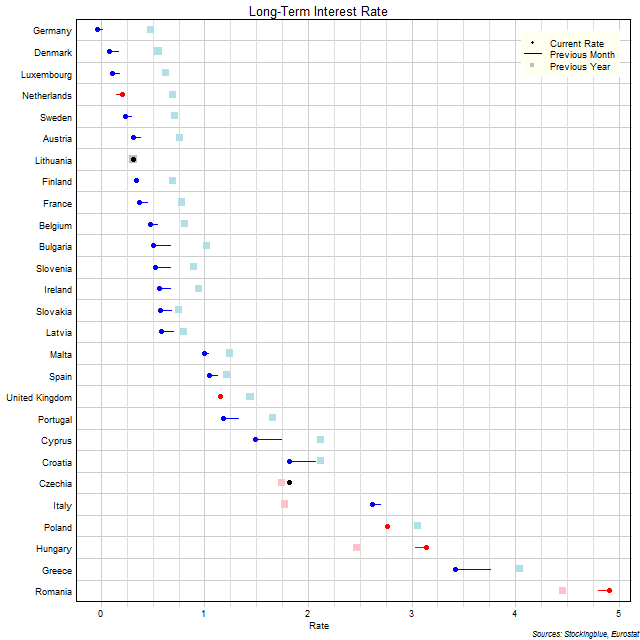The chart above shows the ten-year interest rate in each EU state as of April 2019, the change from the previous month, and the rate one year prior.  Germany is the only state with a negative interest rate.